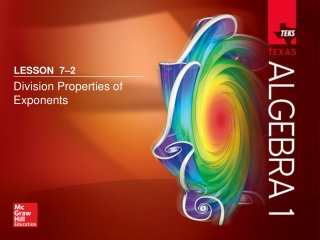DownloadDownload PresentationLESSON 7 –2

# LESSON 7 –2

Download Presentation## LESSON 7 –2

- - - - - - - - - - - - - - - - - - - - - - - - - - - E N D - - - - - - - - - - - - - - - - - - - - - - - - - - -
##### Presentation Transcript

1. LESSON 7–2 Division Properties of Exponents

2. Five-Minute Check (over Lesson 7–1) TEKS Then/Now New Vocabulary Key Concept: Quotient of Powers Example 1: Quotient Powers Key Concept: Power of a Quotient Example 2: Power of a Quotient Key Concept: Zero Exponent Property Example 3: Zero Exponent Key Concept: Negative Exponent Property Example 4: Negative Exponents Example 5: Real-World Example: Apply Properties of Exponents Lesson Menu

3. Determine whether –5x2 is a monomial. Explain your reasoning. A. Yes, the expression is a product of a number and variables. B. No, it has a variable. 5-Minute Check 1

4. Determine whether x3 – y3 is a monomial. Explain your reasoning. A. Yes, the exponents are the same power. B. No, the expression is the difference between two powers of variables. 5-Minute Check 2

5. Simplify (3ab4)(–a4b2). A. 3a5b6 B. –3a5b6 C. 3a3b2 D. 9a3b6 5-Minute Check 3

6. Simplify (2x5y4)2. A. 2x7y6 B. 2x10y8 C. 4x10y8 D. 4x7y6 5-Minute Check 4

7. A. B.10n5 C.5n6 D.5n5 units2 units2 units2 units2 Find the area of the parallelogram. 5-Minute Check 5

8. What is the product (–3x2y3z2)(–17x3z4)? A. 20x5y3z6 B. 20x6y3z8 C. 51x5y3z6 D. 51x6y3z8 5-Minute Check 6

9. Targeted TEKS A.11(B) Simplify numeric and algebraic expressions using the laws of exponents, including integral and rational exponents. Mathematical Processes A.1(E), A.1(G) TEKS

10. You multiplied monomials using the properties of exponents. • Divide monomials using the properties of exponents. • Simplify expressions containing negative and zero exponents. Then/Now

11. zero exponent • negative exponent • order of magnitude Vocabulary

12. Concept

13. Quotient of Powers Group powers that have the same base. Quotient of Powers = xy9 Simplify. Answer:xy9 Example 1

14. A. B. C. D. Example 1

15. Concept

16. Power of a Power Answer: Power of a Quotient Power of a Quotient Power of a Product Example 2

17. Simplify Assume that p and q are not equal to zero. A. AnsA B. AnsB C. AnsC D. AnsD Example 2

18. Concept

19. A. Zero Exponent Answer: 1 Example 3

20. Zero Exponent B. a0 = 1 Simplify. = nQuotient of Powers Answer:n Example 3

21. A. Simplify . Assume that z is not equal to zero. A. B.1 C.0 D.–1 Example 3

22. B. Simplify . Assume that x and k are not equal to zero. A. B. C. D. Example 3

23. Concept

24. A. Simplify . Assume that no denominator is equal to zero. Answer: Negative Exponents Negative Exponent Property Example 4

25. Negative Exponents Group powers with the same base. Quotient of Powers and Negative Exponent Property Example 4

26. Negative Exponents Simplify. Negative Exponent Property Multiply. Answer: Example 4

27. A. Simplify . Assume that no denominator is equal to zero. A. B. C. D. Example 4

28. B. Simplify . Assume that no denominator is equal to zero. A. AnsA B. AnsB C. AnsC D. AnsD Example 4

29. Apply Properties of Exponents SAVINGS Darin has \$123,456 in his savings account. Tabo has \$156 in his savings account. Determine the order of magnitude of Darin’s account and Tabo’s account. How many orders of magnitude as great is Darin’s account as Tabo’s account? Analyze We need to find the order of magnitude of the amounts of money in each account. Then find the ratio of Darin’s account to Tabo’s account. Formulate Round each dollar amount to the nearest power of ten. Then find the ratio. Example 5

30. The ratio of Darin’s account to Tabo’s account is or 103. Apply Properties of Exponents Determine The amount in Darin’s account is close to \$100,000. So, the order is 105. The amount in Tabo’s account is close to 100, so the order of magnitude is 102. Answer: So, Darin has about 1000 times as much as Tabo, or Darin has 3 orders of magnitude as much in his account as Tabo. Example 5

31. Justify The ratio of Darin’s account to Tabo’s account is ≈ 792. The power of ten closest to 792 is 1000, which has an order of magnitude of 103. Apply Properties of Exponents EvaluateRounding and analyzing the orders of magnitude of a solution is an effective tool to determine the reasonableness of a solution. Our solution is reasonable because the actual savings ratio rounds to the order of magnitude. Example 5

32. A circle has a radius of 210 centimeters. How many orders of magnitude as great is the area of the circle as the circumference of the circle? A. 101 B. 102 C. 103 D. 104 Example 5

33. LESSON 7–2 Division Properties of Exponents